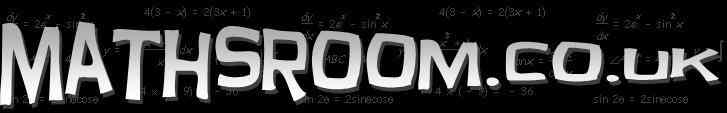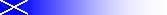Online interactive resources for the Scottish Maths curriculum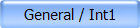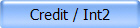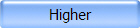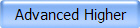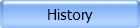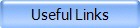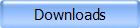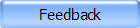5-14 Level D-F Number, Money & Measure - Shape, Position & Movement - Information Handling Number, Money & Measurement Large On-Screen Calculator (Flash) Teaching/Demo Basic functions only. BIG Calculator! (Java Applet) Teaching/Demo Can show up to 80 digits!! (Despite the warning on the page, it works fine!) Base 10 Blocks (Java Applet) Teaching/Demo Exploring whole & decimal numbers, place value with Base 10 Blocks. Includes lesson plan. Cuisenaire Rods (Java Applet) Teaching/Demo Set of moveable rods. Includes lesson plan and pupil activities. 1-100 Square plus Counters (Flash) Teaching/Demo Numeracy tool with moveable counters.Number Grid (Flash) Teaching/Demo 100 square - click to highlight individual numbers. Multiplication Square (Flash) Teaching/Demo 100 square with moveable yellow bars to cover different groups of numbers. 5-14 Assessment - Level D & E Mental Test Practice From the McLaren High School Maths Dept (Select S1-S2 from the home page menu and scroll down the page) Mental Maths Tester (Flash) Practice basic arithmetic mentally. Guess My Number (Shockwave) Find the mystery number by following clues involving even/odd, divisibility, square, prime, etc. Add It Up (Shockwave) Race against clock to click numbers that add up to given value. Count On Convict (Flash) Help the prisoners escape by calculating differences. Estimating (Flash) Estimate values on numberlines. 8 levels from whole numbers to decimals (tenths and hundredths).Positive & Negative Numbers 1 (Shockwave) Order positive and negative numbers. Positive & Negative Numbers 2 (Flash)  Move your spaceship up and down using positve & negative numbers to find all the pods. Functions (Flash)  Colourful function machine game. Some functions involve negative answers. Size & Scale (Flash)  Resize the jigsaw pieces to complete the puzzle. Factors and Primes (Flash) Answer questions on factors and primes by shooting down the correct answer. Interactive Algebra Worksheet Substitute values into expressions and evaluate. Working should be done on paper and answer inputed for correction. Solving Equations Practice Level E-F Solve randomly generated equations, for example: 2x = -6, 5(x + 2) = 15, 4x - 1 = -x + 9. Working should be done on paper and answer inputed for correction. Scientific Notation (Flash) Level F Explore the components of scientific notation. Includes exercises on converting between forms.Fractions 1a Identifying fractions of coloured shapes. 12 pages getting progressively harder. Click ‘next’ at bottom of screen to advance. Fractions 1b Further 4 pages. Fractions 2a Identifying fraction matching position on a number line. Click ‘next’ at bottom of screen to advance. Fractions 2b More pages. Fractions 3a Simplifying Fractions Click ‘next’ at bottom of screen to advance. Fractions 3b Further 2 pages. Fractions 4a Equivalent Fractions 2 pages, click ‘next’ at bottom of screen to advance. Fractions 4b Another 2 pages. Fractions 5 Calculating fractions of quantities. 2 pages, click ‘next’ at bottom of screen to advance. Fractions 6 (Java Applet) Fraction Bars Set up bars to show equivalence, including decimals and percentages. Fractions 7 (Shockwave) Fraction Frenzy Match Equivalent Fractions against the clock. Fractions 8 (Flash) Converting decimals to fractions game Enter correct answer to unlock the tank and shoot the target. Fractions 9 (Java Applet) Fraction Four 2 player Connect4 game with questions on fractions, decimals and percentage conversion. Fractions 10 (Flash) Practice, equivalent, reducing, improper & mixed numbers, multiply & divide, add and subtract fractions. Fractions 11 (Flash) Equivalent Fractions. Match fractions to pictures of fractions.Shape, Position & Movement Match Shapes (Flash) Quick reactions and hand-eye coordination needed to match the shapes for maximum points against the clock. Shape Properties (Flash) Group shapes by properties. 3D-shapes (Java Applet) Teaching/Demo Every 3D shape that you could possibly think of. All 433 of them!! Geometry (Java Applet) Circle, triangles, quadrilaterals, triangles, symmetry. Tiling (Java Applet) Teaching/Demo/Pupil activity Make tilings from a variety of shapes. Angles (Flash) Learn how to measure angles; recognise right, acute and obtuse angles. What's My Angle (Flash) Resource for teaching how to measure angles. Angle Facts (Flash) Interactive investigation of 20 angle facts using an on-screen protractor. Area & Perimeter of Rectangle & Square (Flash) Explore the effects on area & perimeter by changing the lengths of the sides. Area Explorer (Java Applet) Set the perimeter then calculate the area of the shape. Perimeter Explorer (Java Applet) Set the area then calculate the perimeter of the shape. Coordinates 1 (Flash) Direct Billy Bug to the coordinate to get food – first quadrant. (Level D) Coordinates 2 (Flash) Direct Billy Bug to the coordinate to get food - 4 quadrants. (Level E) Coordinates 3 (Shockwave) Correctly plot given coordinate - first quadrant. (Level D) Coordinates 4 (Shockwave) Correctly identify coordinate of points indicated - first quadrant. (Level D) Coordinates 5 Navigate safely through the minefield using coordinates in first quadrant. (Level D) Coordinates 6 Navigate safely through the minefield using coordinates in all four quadrants. (Level E) Discovering The Value Of Pi (Java Applet) Interactive on-line lesson Measure the diameter & cicrcumference and estimate their relationship. Includes lesson plan. Pythagoras Theorem (Flash) Level F Observe the the square on the hypotenuse as you alter the other two sides. Includes interactive exercise and on-line scientific calculator.Information Handling Histogram  Bar Graph  Pie Chart (Java Applet) Stem & Leaf Diagram (Level F) (Java Applet) View and create these statistical diagrams using built in data or user defined data.